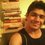# Can you tell me what's wrong with it ?

With no doubts in your mind, i can write :

$\sqrt{-1}=\sqrt{-1}$

[$\sqrt{-1}=i$ i.e. iota]

=> $\sqrt{\frac{-1}{1}}=\sqrt{\frac{1}{-1}}$

=> $\frac{\sqrt{-1}}{\sqrt{1}}=\frac{\sqrt{1}}{\sqrt{-1}}$

=> $\frac{i}{1}=\frac{1}{i}$

cross multiplying

=> $i^{2}=1$

=> $\boxed{-1=1}$ (Because we know $i^{2}=-1$)Note by Sandeep Bhardwaj
6 years, 8 months ago

This discussion board is a place to discuss our Daily Challenges and the math and science related to those challenges. Explanations are more than just a solution — they should explain the steps and thinking strategies that you used to obtain the solution. Comments should further the discussion of math and science.

When posting on Brilliant:

• Use the emojis to react to an explanation, whether you're congratulating a job well done , or just really confused .
• Ask specific questions about the challenge or the steps in somebody's explanation. Well-posed questions can add a lot to the discussion, but posting "I don't understand!" doesn't help anyone.
• Try to contribute something new to the discussion, whether it is an extension, generalization or other idea related to the challenge.
• Stay on topic — we're all here to learn more about math and science, not to hear about your favorite get-rich-quick scheme or current world events.

MarkdownAppears as
*italics* or _italics_ italics
**bold** or __bold__ bold
- bulleted- list
• bulleted
• list
1. numbered2. list
1. numbered
2. list
Note: you must add a full line of space before and after lists for them to show up correctly
paragraph 1paragraph 2

paragraph 1

paragraph 2

[example link](https://brilliant.org)example link
> This is a quote
This is a quote
    # I indented these lines
# 4 spaces, and now they show
# up as a code block.

print "hello world"
# I indented these lines
# 4 spaces, and now they show
# up as a code block.

print "hello world"
MathAppears as
Remember to wrap math in $$ ... $$ or $ ... $ to ensure proper formatting.
2 \times 3 $2 \times 3$
2^{34} $2^{34}$
a_{i-1} $a_{i-1}$
\frac{2}{3} $\frac{2}{3}$
\sqrt{2} $\sqrt{2}$
\sum_{i=1}^3 $\sum_{i=1}^3$
\sin \theta $\sin \theta$
\boxed{123} $\boxed{123}$

## Comments

Sort by:

Top Newest

you cannot write root(a) root(b) as root(ab) if a and b are both negative numbers,,,

certain laws of exponents holds only for positive real numbers,, and why? specifically because it leads to contradictions as root(-1) root (-1) = root(-1*-1)=root(1)=1 which is not true,,,

instead we write it as

$\sqrt { -a } \sqrt { -b } \quad =\quad i\sqrt { a } i\sqrt { b } =-\sqrt { ab } \quad \quad and\quad not\quad \sqrt { (-1)(-1)ab } =\sqrt { ab } \\$ you did the same contradiction in a rather elongated fashion and i can show that your way is same as the one i pointed above,,

Look at the 2nd step.... the result you obtained by exchanging signs among roots is a logical fallacy,

instead you should write

$\frac { \sqrt { -a } }{ \sqrt { b } } =\frac { i\sqrt { a } }{ \sqrt { b } } =-\frac { \sqrt { a } }{ i\sqrt { b } } =\quad -\frac { \sqrt { a } }{ \sqrt { -b } } \\ \\ and\quad then\quad u\quad correctly\quad get\quad -1=-1\quad all\quad back\quad again\\ \\$

- 6 years, 8 months ago

Log in to reply

ure right ! ....... great mathematician LEONHARD EULER did the same mistake once! {of course in a slightly different manner}

- 6 years, 6 months ago

Log in to reply

I'm searching for a rigorous fault, and I could find some possibilities. I don't like $\sqrt{-1}$ being replaced by $\iota$, cuz $\iota = \pm \sqrt{-1}$, though I may be wrong. There may be something extraneous done while playing with the square roots and squares.

- 6 years, 8 months ago

Log in to reply

If I say that

$\sqrt{\frac{a}{b}}=\frac{\sqrt{a}}{\sqrt{b}}$ , only if a and b are both positive or both negative...

And if exactly one of a and b is positive, then

$\sqrt{\frac{a}{b}}=i. \frac{\sqrt{|a|}}{\sqrt{|b|}}$.

You can say that i am trying to make a new rule , which would give its best to control some functionings of such expressions.

One rule I know is $\sqrt{a}.\sqrt{b}=\sqrt{a.b}$ ; if and only if at least one of a and b is non-negative. And the second rule may be which i discussed above :P

- 6 years, 8 months ago

Log in to reply

So is the rule stated by you true or not ? I have read about the latter but not the first one.

- 6 years, 7 months ago

Log in to reply

I hope so that it's true. But i ain't 100% sure.!

- 6 years, 7 months ago

Log in to reply

Isn't$\sqrt {\frac{a}{b}} =\frac {\sqrt {a}}{\sqrt {b}}$ possible when a and b are positive

- 6 years, 8 months ago

Log in to reply

Offcourse, you can write it ; if a and b are both positive. But what in case of above contradiction ?

- 6 years, 8 months ago

Log in to reply

I am saying that you can split the term only if the statement i said is true then your 3 rd step will be wrong

- 6 years, 8 months ago

Log in to reply

Ok . So is there any RULE which says that $\sqrt{\frac{a}{b}}=\frac{\sqrt{a}}{\sqrt{b}}$ , if and only if both a and b are positive. It is true for both negatives too. And also true in one way :if one of them is positive and the other one is negative, for eg : $\sqrt{\frac{-1}{2}}=\frac{\sqrt{-1}}{\sqrt{2}}=i.\frac{\sqrt{1}}{\sqrt{2}}$ . Here i denotes iota.

- 6 years, 8 months ago

Log in to reply

Roots with complex donot follow distributive law of algebra

- 5 years, 4 months ago

Log in to reply

×

Problem Loading...

Note Loading...

Set Loading...